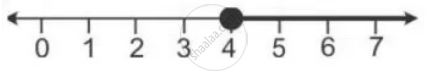Share

Given: a = {X: 11x – 5 > 7x + 3, X ∈ R} and B = {X: 18x – 9 ≥ 15 + 12x, X ∈ R}. Find the Range of Set a ∩ B and Represent It on the Number Line. - ICSE Class 10 - Mathematics

ConceptRepresentation of Solution on the Number Line

Question

Given:
A = {x: 11x – 5 > 7x + 3, x ∈ R} and
B = {x: 18x – 9 ≥ 15 + 12x, x ∈ R}.
Find the range of set A ∩ B and represent it on the number line.

Solution

A = {x: 11x – 5 > 7x + 3, x ∈ R}

= {x: 4x > 8, x ∈ R}

= {x: x > 2, x ∈ R}

B = {x: 18x – 9 ≥ 15 + 12x, x ∈ R}

= {x: 6x ≥ 24, x ∈ R}

= {x: x ≥ 4, x ∈ R}

A ∩ B = {x: x ≥ 4, x ∈ R}

It can be represented on number line as:Is there an error in this question or solution?

APPEARS IN

Solution Given: a = {X: 11x – 5 > 7x + 3, X ∈ R} and B = {X: 18x – 9 ≥ 15 + 12x, X ∈ R}. Find the Range of Set a ∩ B and Represent It on the Number Line. Concept: Representation of Solution on the Number Line.
S